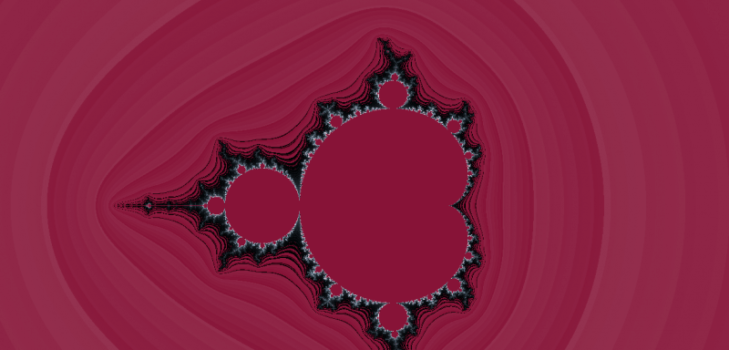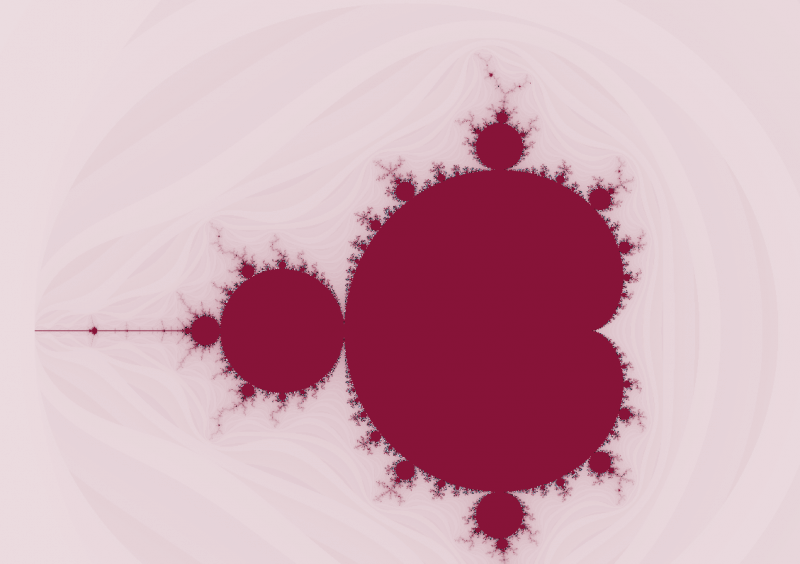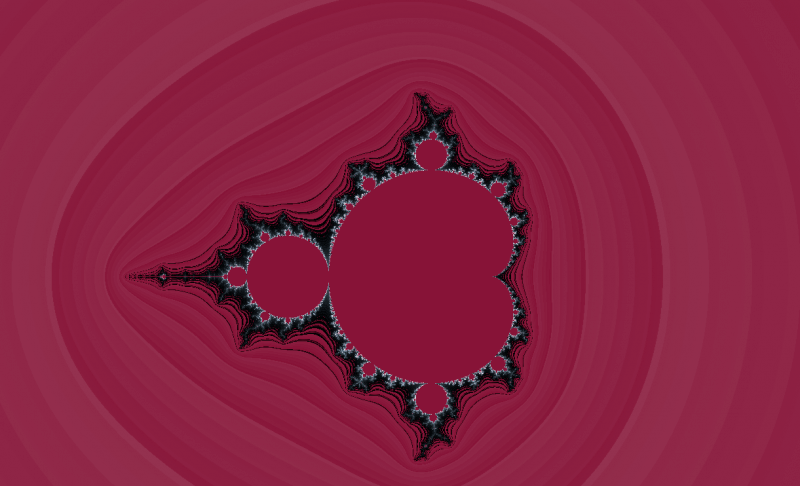# Generate A Mandelbrot Set In H2OBy H2O.ai Team | minute read | February 15, 2014

Category: UncategorizedRoses are red,
Violets are ~ Blue,
H2O is sweet,
And fractals are too!$$z_n = z_{n-1}^P + c$$


Where c is a “candidate” complex number. (Typically you’ll see $$P = 2$$ — that’s what we’ll do too).
We set the the size of the sequence to the number of iterations we want, and measure convergence by looking at the modulus of $$z_n$$ at each iteration. If the modulus exceeds some threshold, we say the sequence diverges and “c” is then not in the Mandelbrot set, otherwise it converges!
The point “c” is then colored according to the number of iterations accomplished. To produce really stellar images, it’s better to have fine-grained color gradients in your palette and then alpha compost them. The palette we used to generate the Valentine’s day grams in this post had ~200 colors. Although there is still some “banding”, the gradients look pretty smooth on my screen (Retina 15″ MBP).
OK, let’s get to work!

### First Glance

At first glance, there are a few important inputs:

Image dimensions - Height and Width of image in pixels. (We generated data by hand)<br />
Max Iterations - Iterations of the recurrence relation.<br />
Convergence Threshold - What constitutes membership in the Mandelbrot set.<br />


Here are a few more that we may want later when we’re exploring our final images:

Zoom - Zoom in on the Mandelbrot set at the (offsetX,offsetY) position.
offsetX - Zoom focus x-coordinate.
offsetY - Zoom focus y-coordinate.


The goal is to add a Mandelbrot UI for setting the input parameters and upon submitting produces the Mandelbrot image.
First let’s start off with a skeleton implementation and fill it in a little bit at a time:

/**
* The main class that holds all of the Mandelbrot-related functionality.
* Any UI page must subclass Request2 and implement Response.serve().
* This is demonstrated below.=
*/
public class Mandelbrot extends Request2 {
/**
* Declare any public API arguments here.
* Here's where our inputs will go: Height, Width, Threshold,
* Max Iterations, Zoom, OffsetX, OffsetY
*/
@Override protected Response serve() {
// This is where we'll make the call to generate the Mandelbrot
// set & produce the image.
}
/**
* A class that represents our fractal.
* This is the centerpiece of our implementation.
* To do distributed & parallel work, we will have this
* class extend MRTask2 and override the map() method.
* More on this below....
*/
protected static class FractalSet extends MRTask2<FractalSet> {
private double[] z_squared(double a, double b) {
//return the square of the complex number z = a + ib
}
private double modulus(double a, double b) {
//return the modulus of a complex number z = a + ib
}
public int iterate(double a, double b) {
// take the candidate complex number c = a + ib
// and iterate with the recurrence relation zn = z_{n-1}^2 + c
// return the color
}
private int palette(int iters) {
//choose color from palette based on the number of iterations
//Color returned is number of iterations modulo palette size
}
private int linerp(int color1, int color2, double alpha) {
//linear interpolate (AKA alpha compost) two colors.
}
@Override public void map(Chunk[] cs, NewChunk[] ncs) {
/**
* Break the input into chunks and compute the
* the iteration number per row (i.e. per pixel)
* Produce a new frame with x, y, and decimal color.
*/
}
}
public class MandelbrotView extends JFrame {
/**
* Here we set the up details of the JFrame.
* We use Java's BufferedImage to make the final image
* This part is single threaded...
*/
}
}


Starting with the FractalSet class, we can knock down the z_squared and modulus methods pretty easily:
The square of a complex number can be computed using the FOIL method, and remembering that the imaginary unit follows the same squaring rules as real numbers:

$$z^2 = (a + bi) * (a + bi) = (a^2 - b^2) + i*2*a*b$$


The modulus is a fancy name for length, or magnitude, or distance with respect to the origin. So:

$$|z| = \sqrt(a*a + b*b)$$


Our methods are written as follows:

private double[] z_squared(double a, double b) {
double newa = a*a - b*b;
double newb = 2*a*b;
return new double[]{newa, newb};
}
private double modulus(double a, double b) {
return Math.sqrt(a*a + b*b);
}


The palette is also pretty simple to implement. We chose colors suitable for the day, and there 193 of them. Again we chose colors according to the number of iterations taken modulo the number of colors in our palette:

private int palette(int it) {
int[] color_palette = new int[]{7473449,7539499,7605805,7737391,7803697,7935284,8001590,8133176,8199482,8331325,8397375,8529217
,8595267,8727109,8793160,8925002,8991308,9057358,9189201,9255251,9387093,9453143,9584986,9651036,9782878,9849184,9980770,
10047077,10178663,10244969,10376555,10442862,10574704,10640754,10707060,10838647,10904953,11036539,11102845,11234431,11300738,
11432580,11498630,11630472,11696523,11828365,11894415,12026257,12092564,12158614,12290456,12356506,12488348,12554399,12686241,12752291,
12884133,12950440,13082026,13148332,13279918,13346224,13477811,13544117,13675959,13742009,13808316,13939902,14006208,14137794,14204101,14335687,
14401993,14533835,14599885,14731728,14797778,14929620,14995670,15127513,15193819,15259869,15391711,15457762,15589604,15655654,15787496,15853546,
15985389,16051695,16183281,16249587,16381174,16447480,16579066,16645372,10799852,10668009,10536423,10404836,10338786,10207199,10075613,10009562,
9877976,9746389,9614547,9548496,9416910,9285324,9219273,9087687,8956100,8824514,8758463,8626877,8495034,8428984,8297397,8165811,8099761,7968174,
7836588,7705001,7638951,7507108,7375522,7309471,7177885,7046298,6914712,6848661,6717075,6585489,6519438,6387596,6256009,6124423,6058372,5926786,
5795199,5729149,5597562,5465976,5399926,5268083,5136497,5004910,4938860,4807273,4675687,4609636,4478050,4346463,4214621,4148570,4016984,3885398,
3819347,3687761,3556174,3424588,3358537,3226951,3095108,3029058,2897471,2765885,2699835,2568248,2436662,2305075,2239025,2107182,1975596,1909545,
1777959,1646372,1514786,1448735,1317149,1185563,1119512,987670,856083,724497,658446,526860,395273,329223,197636,66050};
return color_palette[it % color_palette.length];
}


In order to compost two colors together, we’ll use a linear interpolation function that takes two colors, strips out their rgb components, blends the components and then combines the r, g, b colors into a single int value.

private int linerp(int color1, int color2, double alpha) {
int r1 = (color1 >> 16) & 0xFF;
int g1 = (color1 >> 8) & 0xFF;
int b1 = color1 & 0xFF;
int r2 = (color2 >> 16) & 0xFF;
int g2 = (color2 >> 8) & 0xFF;
int b2 = color2 & 0xFF;</p>
int rgb = (int)Math.round(r1*alpha + r2*(1 - alpha));
rgb = (rgb << 8) + (int)Math.round(g1*alpha + g2*(1 - alpha));
rgb = (rgb << 8) + (int)Math.round(b1*alpha + b2*(1 - alpha));
return rgb;
}


Let’s tackle the iterate()function now.
The idea here is to generate a new complex number while it does not exceed some threshold and the number of iterations is below some threshold. Additionally, though, we want to produce a color from this process, and attempt to smooth the color transitions caused by different numbers of iterations. Therefore, if the number of iterations reached is not maximal, then we can color using the following pieces of math:
$$\nu = (\log(z_n) / \log(T) ) / \log(T)$$
where $$T$$ is the threshold. By subtract this from the iteration count and then flooring it, we can retrieve a color from our palette. Retrieve the neighboring color from the palette and then compost them. Here’s what that code looks like:

public int iterate(double a, double b) {
int n = 0;
double it;
double ca = (a - _density/2) / _zoom;
double cb = (b - _density/2) / _zoom;
a = b = 0;
int iter = _maxIters;
while((modulus(a, b) < _threshold) && (iter > 0)) {
double[] z2 = zsquared(a,b);
a = z2 + ca;
b = z2 + cb;
iter--;
}
if ( iter > 0) {
double zn = modulus(a, b);
double nu = ( Math.log(zn) / Math.log(_threshold) ) / Math.log(_threshold);
it = iter + 1 - nu;
} else it = iter;
int _it = (int)Math.floor(it);
int color1 = palette(_it);
int color2 = palette(_it + 1);
return linerp(color1, color2, it % 1);
}


The last piece of this is the map. It takes a chunk array, which is a chunk of rows, and produces some new chunks. The coder doesn’t need to worry about how the chunks are managed, H2O takes care of all that.
The chunk array is expected to have length 2, since we are working with 2 columns of input (x-dim, y-dim). The output dimension is 3: x, y, color. Again, color is the output from the iterate() function above. Here’s what the map looks like:

@Override public void map(Chunk[] cs, NewChunk[] ncs) {
assert cs.length == 2;
for(int row = 0; row < cs._len; row++) {
double x = cs.at0(row);
double y = cs.at0(row);
int color = iterate(x,y);
}
}


### @Override Response serve()

Let’s work on the serve() call as our last part (the JFrame part is standard Java, I’ll just hand that out at the end. No secret sauce there.)
There’s a standard “header” to each Request2 subclass that contains various UI knobs and declarations.
Here’s what a starting header might look like (OffsetX and OffsetY are left to the reader!):

public class Mandelbrot extends Request2 {
static final int API_WEAVER = 1; // This file has auto-gen'd doc & json fields
static public DocGen.FieldDoc[] DOC_FIELDS; // Initialized from Auto-Gen code.
@API(help = "Destination key", required = false, filter = Default.class)
protected final Key destination_key = Key.make("Mandelbrot");
@API(help = "Data frame", required = true, filter = Default.class)
public Frame source;
@API(help = "Threshold to stop iterating.", required= true, filter = Default.class)
public int threshold = 2;
@API(help = "Max Iterations", required = true, lmin = 1, filter = Default.class)
public int max_iters = 10000;
@API(help = "Zoom in on Mandelbrot drawing (default is no zoom)", required = false, lmin = 1, filter = Default.class)
public int zoom = 1;
......


The serve call will instantiate a new FractalSet object (constructor provided in full code) and that will invoke the computation with the function doAll(int, Vec[]), which takes an integer (the number of output fields) and a Vec array. A Vec is an internal H2O data format representing a single column vector . The output frame is then retrieved and passed on to a MandelbrotView object (not shown yet) that creates a new BufferedImage object, loads in the pixels and colors, and finally paints it. Here’s the serve() call code:

@Override protected Response serve() {
try {
FractalSet F = new FractalSet(max_iters, zoom, threshold, source.anyVec().length());
Vec[] vecs = source.vecs();
F.doAll(3, vecs);
Frame fr = F.outputFrame(destination_key, new String[]{"x", "y", "decimal_color"}, null);
fr.delete_and_lock(destination_key).unlock(destination_key);
UKV.put(destination_key, fr);
new MandelbrotView(fr).setVisible(true);
} catch( Throwable t ) {
return Response.error(t);
}
return Inspect2.redirect(this, destination_key.toString()); //MandelbrotView.redirect(this, destination_key);
}


And last but not least, the MandlebrotView class:

public class MandelbrotView extends JFrame {
private final int width = 1500;
private final int height = 1500;
private BufferedImage I;
private Frame _fr;
public MandelbrotView(Frame fr) {
super("Mandelbrot Set");
_fr = fr;
setBounds(100, 100, width, height);
setResizable(false);
setDefaultCloseOperation(DISPOSE_ON_CLOSE);
I = new BufferedImage(getWidth(), getHeight(), BufferedImage.TYPE_INT_RGB);
Vec[] vecs = fr.vecs();
for (int row = 0; row < vecs.length(); row++) {
I.setRGB((int)vecs.at8(row), (int)vecs.at8(row), (int)vecs.at8(row));
}
}
@Override public void paint(Graphics g) {
Graphics2D g2 = (Graphics2D)g;
RenderingHints rh = new RenderingHints(
RenderingHints.KEY_ANTIALIASING,
RenderingHints.VALUE_ANTIALIAS_ON);
RenderingHints rh2 = new RenderingHints(
RenderingHints.KEY_ALPHA_INTERPOLATION,
RenderingHints.VALUE_ALPHA_INTERPOLATION_QUALITY);
RenderingHints rh3 = new RenderingHints(
RenderingHints.KEY_COLOR_RENDERING,
RenderingHints.VALUE_COLOR_RENDER_QUALITY);
RenderingHints rh4 = new RenderingHints(
RenderingHints.KEY_DITHERING, RenderingHints.VALUE_DITHER_ENABLE);
g2.setRenderingHints(rh2);
g2.setRenderingHints(rh);
g2.setRenderingHints(rh3);
g2.setRenderingHints(rh4);
g2.drawImage(I, 0, 0, this);
}
}


Here’s a few more images generated by this code:Happy Valentine’s Day!